# Third Chapter Lesson-4: Conversion among Binary, Octal & Hexadecimal numbers.

## At the end of this lesson-

• 1. You will be able to convert Octal & Hexadecimal to Binary Number system.
• 2. You will be able to convert Binary to Octal & Hexadecimal Number system.
• 3. You will be able to convert Octal to Hexadecimal Number system.
• 4. You will be able to convert Hexadecimal to Octal Number system.

## Conversion among Non-Decimal that means Binary, Octal & Hexadecimal Number Systems:

Step-01: Convert the number from any base to base 10.

Step-02: Convert the number from base 10 to any base.

That is, in case of non-decimal numbers, any conversion can be done in two steps.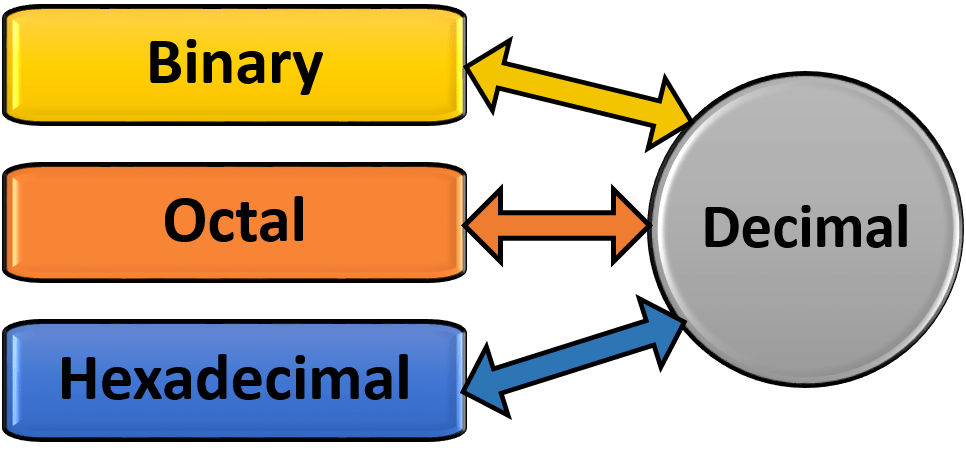### Except above rules there are following rules:

2n (where, n=0,1,2,3,…..) formula can be used to convert directly from Octal & Hexadecimal into Binary and from Binary into Octal & Hexadecimal.

• In case of Octal, 4 2 1 ( 2n ; where n=0,1,2)
• In case of Hexadecimal, 8 4 2 1 ( 2n ; where n=0,1,2,3)

Above rules have been applied in the following conversions-### Conversion of octal number into binary number:

Same rules are followed for integer and fractional part-

Step-1: Convert each octal digit into 3-bit binary number [ follow 4 2 1 rules]

Step-2: Combine the binary groups of all digits.

Example: Convert (375.24)8 into binary number system.

#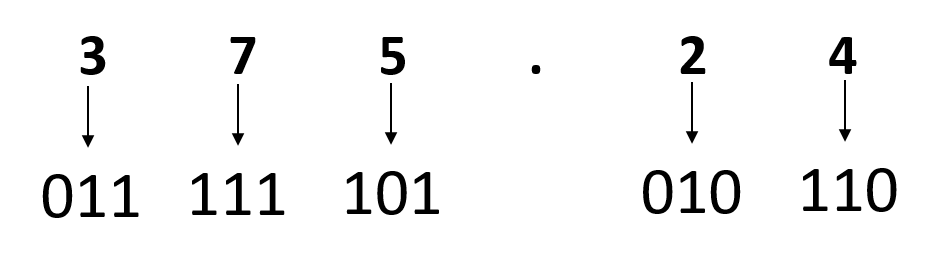So, (375.24)= (011111101.010110)2

• Convert (127)8 into binary number system.
• Convert (.7125)8 into binary number system.

### Conversion of hexadecimal number into binary number:

Same rules are followed for integer and fractional part-

Step-1: Convert each hexadecimal digit into 4-bit binary number [ follow 8 4 2 1 rules]

Step-2: Combine the binary groups of all digits.

Example: Convert (35D.4F)16 into binary number system.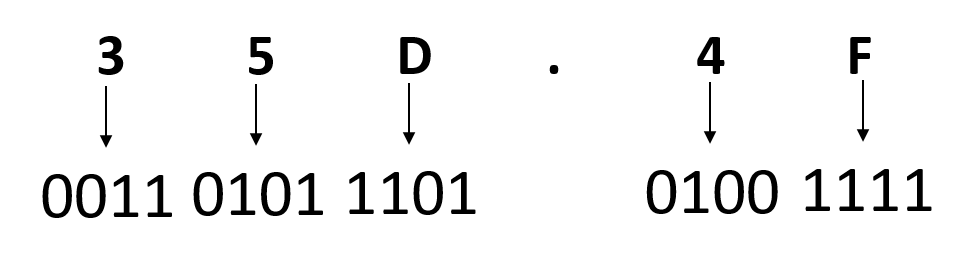So, (35D.4F)16 = (001101011101.01001111)2

• Convert (D218)16 into binary number system.
• Convert (.1C39)16 into binary number system.

### Conversion of binary number into octal number:

#### For integer and  fractional number-

Step-1: In case of integer number divide the binary numbers into groups of  3-bits form right to left and In case of fractional number divide the binary numbers into groups of 3-bits form left to right.

[ In case of integer number, If lack of bits to make 3-bits group, add the required number of zeros on the left and in case of fractions, If lack of bits to make 3-bits group, add the required number of zeros on the right ]

Step-2: Write octal value of each group of 3-bits

Step-3: Combine the octal value

Example: Convert (10101011.1011011)2 into octal number system.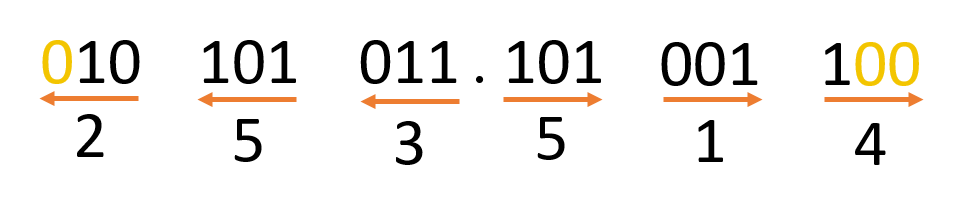So, (10101011.1011011)=(253.514)8

• Convert (1101001)2 into octal number system.
• Convert (.1010011)2 into octal number system.

### Conversion of binary number into hexadecimal number:

#### For integer and  fractional number-

Step-1: In case of integer number divide the binary numbers into groups of  4-bits form right to left and In case of fractional number divide the binary numbers into groups of 4-bits form left to right.

[ In case of integer number, If lack of bits to make 4-bits group, add the required number of zeros on the left and in case of fractions, If lack of bits to make 4-bits group, add the required number of zeros on the right ]

Step-2: Write hexadecimal value of each group of 3-bits

Example: Convert (0111001011.1010011)2 into hexadecimal number system.So, (0111001011.1010011)2 = (1CB.A6)16

• Convert (1101101)2 into hexadecimal number system.
• Convert (.1010011)2 into hexadecimal number system.

### Conversion of Octal into Hexadecimal Number:

The following two ways to convert octal to hexadecimal number-

One: First octal to decimal then decimal to hexadecimal

Two: First octal to binary then binary to hexadecimalExample: Convert (375.246)8 into Hexadecimal number.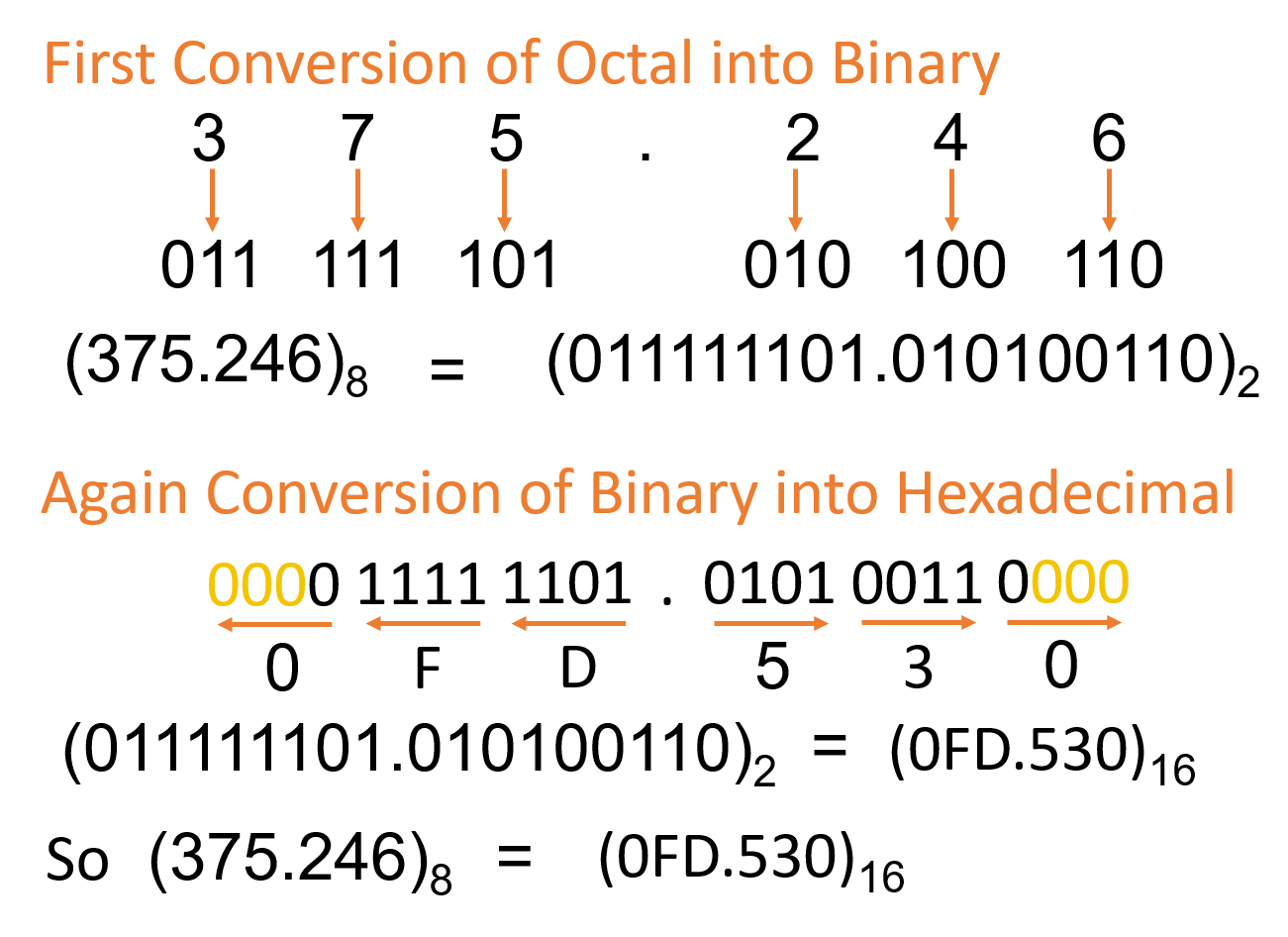• Convert (5273)8 into hexadecimal number.
• Convert (.5137)8 into hexadecimal number.

### Conversion of Hexadecimal into Octal Number:

The following two ways to convert hexadecimal to octal number-

One: First hexadecimal to decimal then decimal to octal

Two: First hexadecimal to binary then binary to octal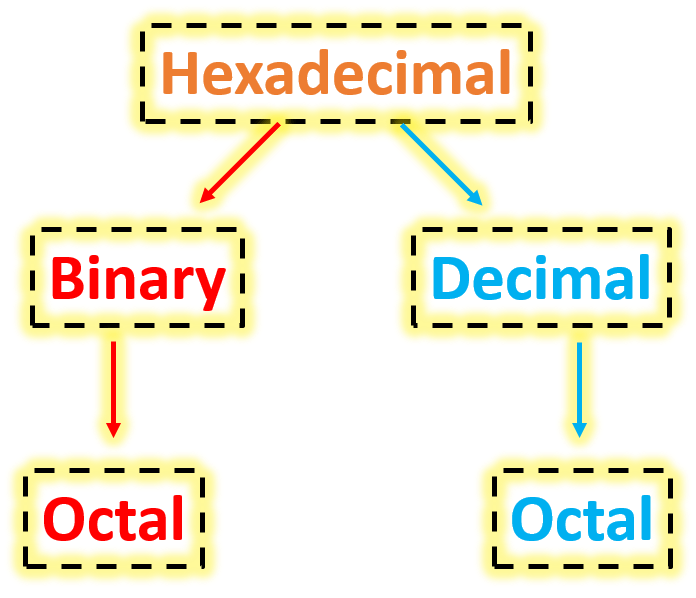Example: Convert (08B.FCD)16 into Octal number.• Convert (5F293)16 into octal number.
• Convert (.A127)16 into octal number.

## Lesson Evaluation-

Knowledge Based Questions:

Comprehension Based Questions:

Creative Questions:

According to the stem answer the following questions:

The professor of ICT was teaching the number system in the classroom. When asked about Imran’s half-yearly and annual test scores for his ICT subject, he said he got (37)8 marks in half-yearly and (3F)16 marks in annual examination. When the other students asked Sir if they did not understand what it meant, Sir explained the details.

c) Convert Imran’s marks obtained in half-yearly and annual exam into binary numbers.

d) Convert Imran’s half-yearly marks into hexadecimal and annual marks into octal.

Multiple Choice Questions:

1. Which one is equivalent binary to (127)8?

a) 1010101  b) 1010111      c) 1110101     d) 1011101

2. Which one is equivalent binary to 4C ?

a) 11001100   b) 01001100     c) 01001010   d) 01001101

3. Which one is equivalent hexadecimal to (1110.0011)?

a) E.3     b) E.8      c) E.C    d) C.E

4. Which one is equivalent hexadecimal to (11011.110111)2 ?

a) 1B.37    b) 1B.DC    c) D8.DC  d) D8.37

5. Equivalent value of (1010)2

i. (10)10 ii. (12)iii. (14)16

Which one is correct?

a) i & ii       b) i & iii        c) ii & iii        d) i,ii & iii

Mr. Atik said to Kamal, ” How old are you?” Kamal replied (101101)2

6. Which one is equivalent octal value to Kamal’s age?

a) (25)8 b) (35)c) (55)d) (65)8

7. How old will Kamal be in binary after ten years?

a) (101011)b) (101110)c) (101111)d) (110111)2

8. Equivalent value of (110110)2

i. (66)ii. (54)10 iii. (36)16

Which one is correct?

a) i & ii       b) i & iii        c) ii & iii        d) i,ii & iii

Written by,